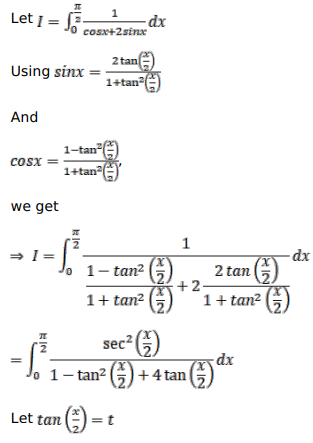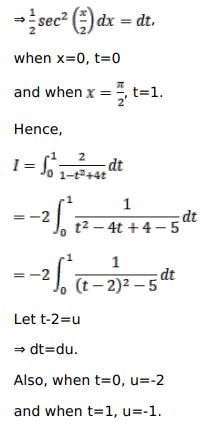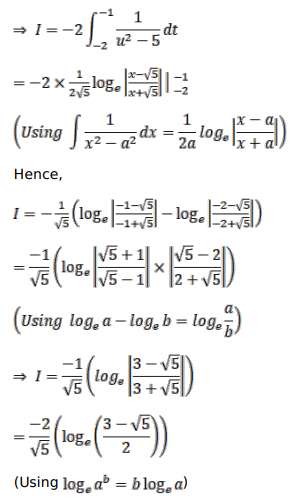# Evaluate the following integralsQuestion:

Evaluate the following integrals

$\int_{0}^{\pi / 2} \frac{d x}{(\cos x+2 \sin x)}$

Solution: